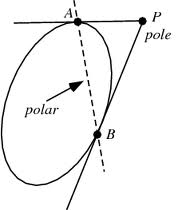# QUESTON 1).The angle between a pair of tangents drawn from a point P to the circle x2 + y2 + 4x - 6y + 9 sin2 + 13cos2 = 0 is 2. The equation of the locus of the point P is1. x2 + y2 + 4x - 6y + 4 = 02. x2 + y2 + 4x - 6y – 9 = 03. x2 + y2 + 4x - 6y - 4 = 04. x2 + y2 + 4x - 6y + 9 = 05 years ago

The equation of the circle is x2 + y2 + 4x - 6y + 9 sin2 +13 cos 2 = 0

Hence, the center of the circle = (-2, 3)

Radius of the circle is v (-2)2 + (32) – 9 sin2 -13cos2

= v13 – 9 sin2 -13cos2

= v13sin2 – 9 sin2

= v4 sin2 = 2 sin

Now, sin = OA/OP = 2 sin / v(h+2)2 + (k-3)2

Hence, (h+2)2 + (k-3)2 = 4

h2 + k2 + 4h -6k +9 = 0.

Therefore, the locus of P is x2 +y2 + 4x - 6y +9 = 0.

5 years agoimage related to the above solution.
For futhur queries, mail me on navneet25799@gmail. com

Navneet Chandan
IIT DELHI

2 years ago

x² + y² + 4x – 6y + 9 sin²α + 13 cos²α = 0

=> x² + 4x + 4 - 4  + y² - 6y  + 9  - 9 + 9 sin²α + 13 cos²α = 0

=> (x +2)² +(y - 3)² - 13 + 9 sin²α + 13 cos²α = 0

=>  (x +2)² +(y - 3)² = 13 - 13 cos²α - 9 sin²α

=> (x +2)² +(y - 3)² =  13Sin²α - 9 sin²α

=>  (x +2)² +(y - 3)² =  4Sin²α

=>   (x +2)² +(y - 3)² =  (2Sinα)²

center = -2 , 3

The angle between a pair of tangents drawn from a point P = 2α

half of 2α = α

=> Sinα = Radius/(distance of P from center of Circle)

=> distance of P from center of Circle = Radius/Sinα

=> distance of P from center of Circle = 2 Sinα/Sinα

=>  distance of P from center of Circle = 2

Distance of P from center

= √(x - (-2))² + (y - 3)²  = 2

Squaring both sides

=> x² + 4x + 4 + y² - 6y + 9 = 4

=> x² + y² + 4x - 6y + 9 = 0